Скачать презентацию Stereo vision A brief introduction Máté István MSc

81177d2fab9595c98f70a5539d502398.ppt

• Количество слайдов: 30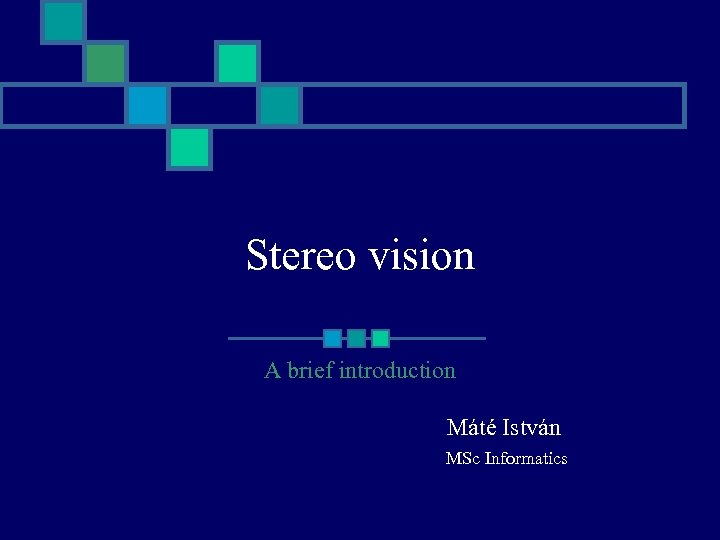Stereo vision A brief introduction Máté István MSc Informatics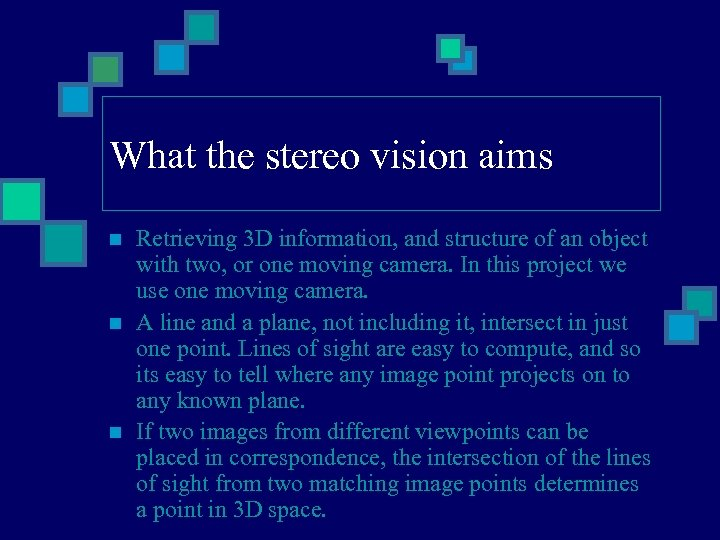What the stereo vision aims n n n Retrieving 3 D information, and structure of an object with two, or one moving camera. In this project we use one moving camera. A line and a plane, not including it, intersect in just one point. Lines of sight are easy to compute, and so its easy to tell where any image point projects on to any known plane. If two images from different viewpoints can be placed in correspondence, the intersection of the lines of sight from two matching image points determines a point in 3 D space.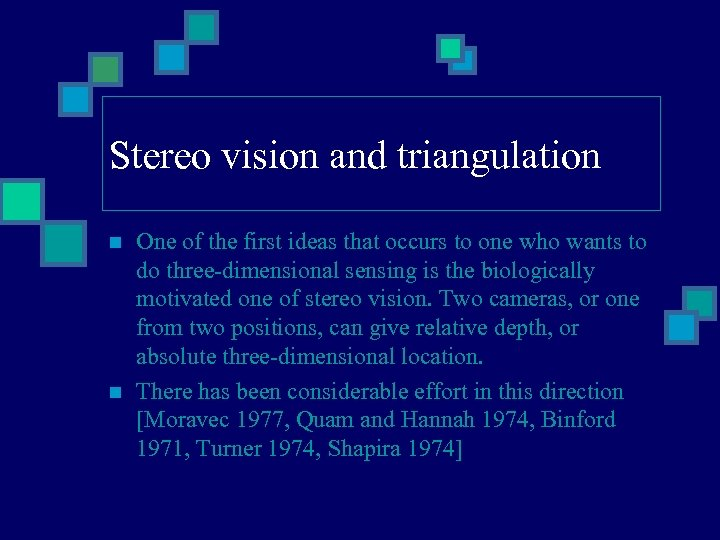Stereo vision and triangulation n n One of the first ideas that occurs to one who wants to do three-dimensional sensing is the biologically motivated one of stereo vision. Two cameras, or one from two positions, can give relative depth, or absolute three-dimensional location. There has been considerable effort in this direction [Moravec 1977, Quam and Hannah 1974, Binford 1971, Turner 1974, Shapira 1974]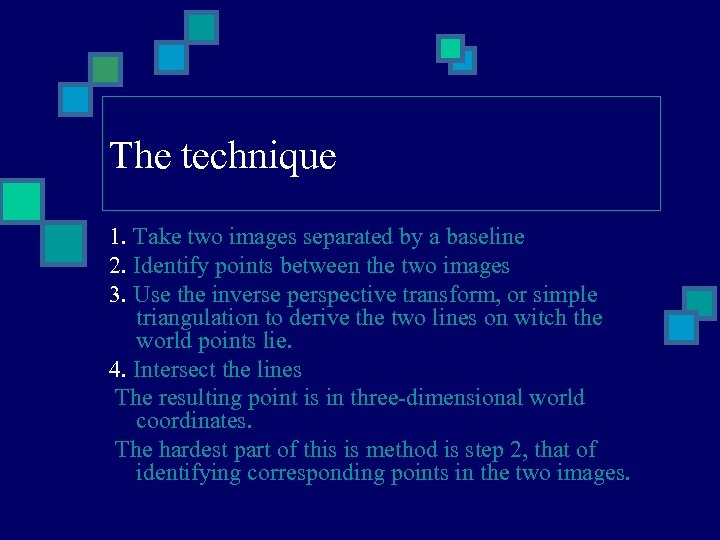The technique 1. Take two images separated by a baseline 2. Identify points between the two images 3. Use the inverse perspective transform, or simple triangulation to derive the two lines on witch the world points lie. 4. Intersect the lines The resulting point is in three-dimensional world coordinates. The hardest part of this is method is step 2, that of identifying corresponding points in the two images.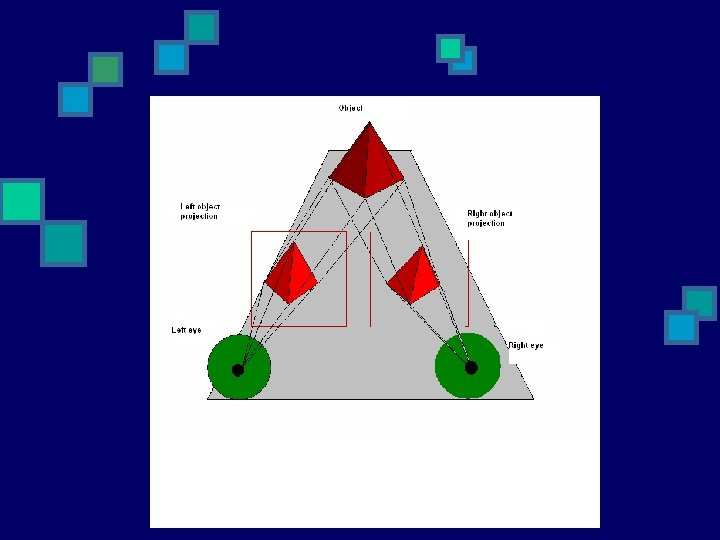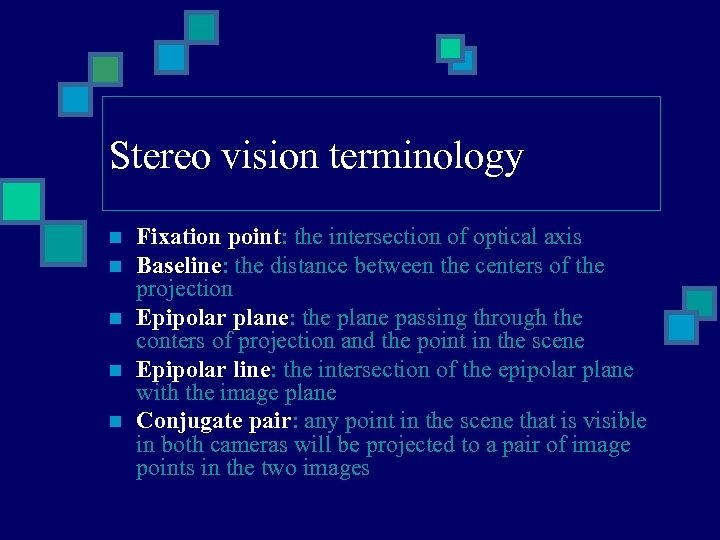Stereo vision terminology n n n Fixation point: the intersection of optical axis Baseline: the distance between the centers of the projection Epipolar plane: the plane passing through the conters of projection and the point in the scene Epipolar line: the intersection of the epipolar plane with the image plane Conjugate pair: any point in the scene that is visible in both cameras will be projected to a pair of image points in the two images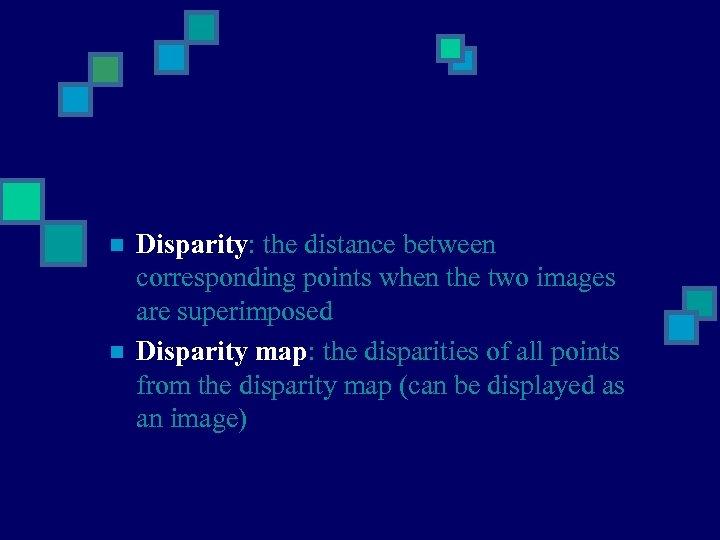n n Disparity: the distance between corresponding points when the two images are superimposed Disparity map: the disparities of all points from the disparity map (can be displayed as an image)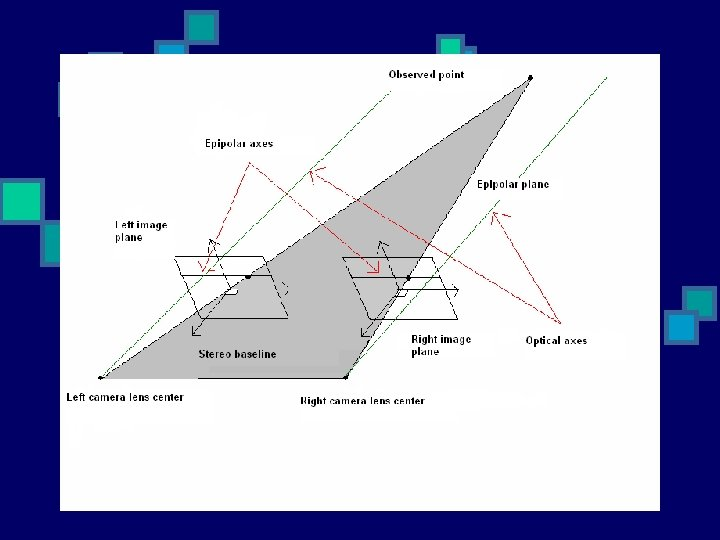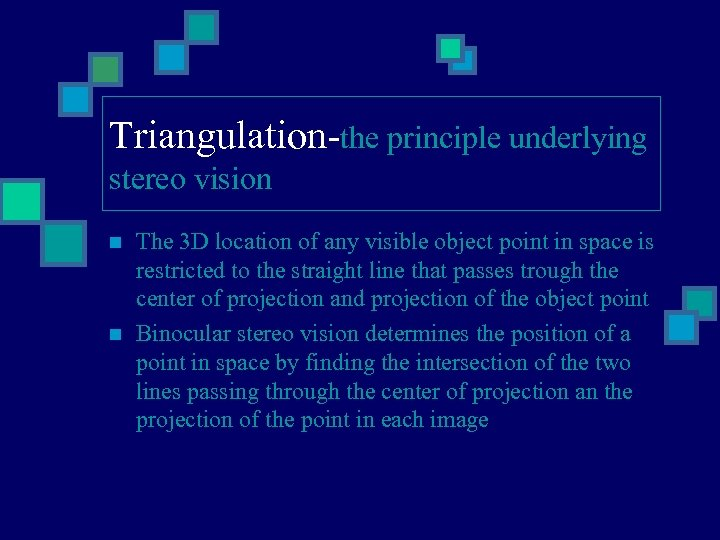Triangulation-the principle underlying stereo vision n n The 3 D location of any visible object point in space is restricted to the straight line that passes trough the center of projection and projection of the object point Binocular stereo vision determines the position of a point in space by finding the intersection of the two lines passing through the center of projection an the projection of the point in each image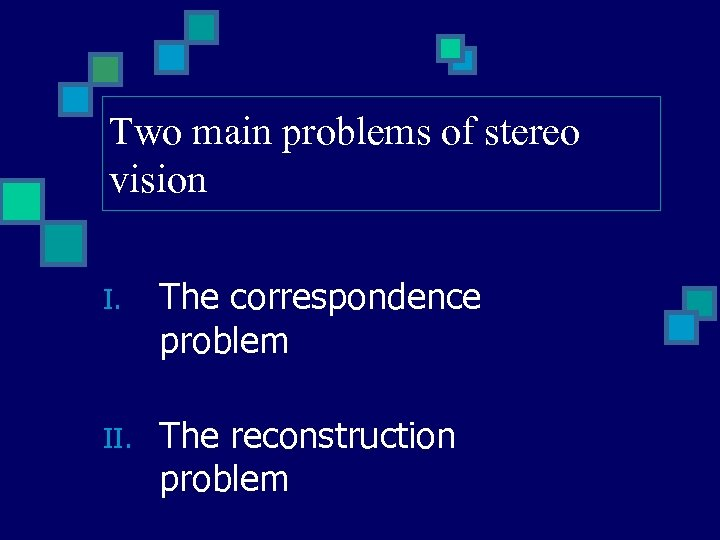Two main problems of stereo vision I. The correspondence problem II. The reconstruction problemI. The correspondence problem Finding pairs of matched points such, that each point in the pair is the projection of the same 3 D point n n Triangulation depends crucially on the solution of the correspondence problem. Ambiguous correspondence between points in the two images may lead to several different consistent interpretation of the scene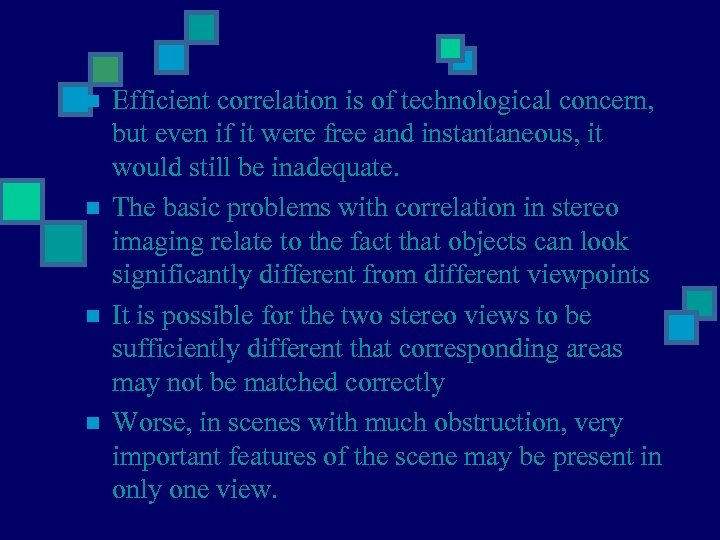n n Efficient correlation is of technological concern, but even if it were free and instantaneous, it would still be inadequate. The basic problems with correlation in stereo imaging relate to the fact that objects can look significantly different from different viewpoints It is possible for the two stereo views to be sufficiently different that corresponding areas may not be matched correctly Worse, in scenes with much obstruction, very important features of the scene may be present in only one view.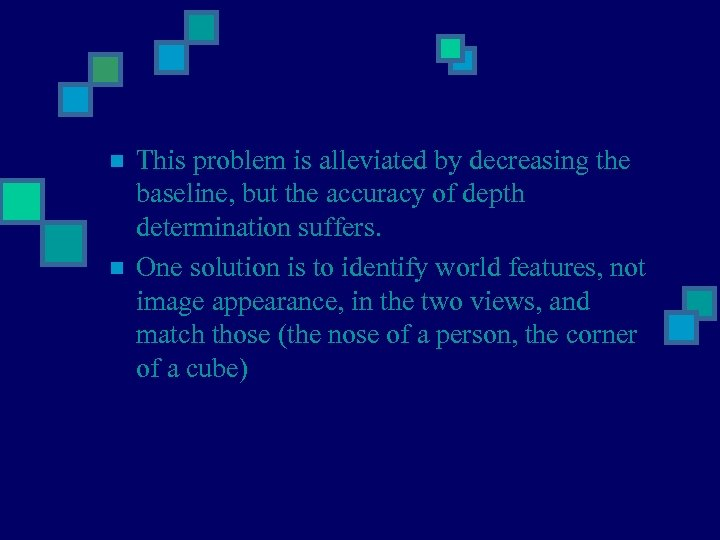n n This problem is alleviated by decreasing the baseline, but the accuracy of depth determination suffers. One solution is to identify world features, not image appearance, in the two views, and match those (the nose of a person, the corner of a cube)Why is the correspondence problem difficult? n n Some points in each image will have no corresponding point in the other image, bacause: The cameras may have different fields of view Due to occlusion A stereo system must be able to determine the image parts that should not be matched.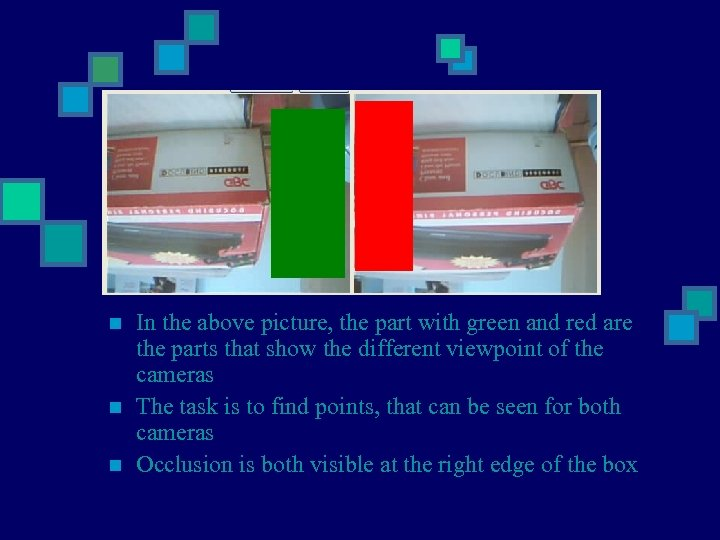n n n In the above picture, the part with green and red are the parts that show the different viewpoint of the cameras The task is to find points, that can be seen for both cameras Occlusion is both visible at the right edge of the boxMethods for establishing correspondence n n n There are two issues to be considered: How to select candidate matches ? How to determine the goodness of a match? A possible class of algorithm Correlation based attempt to establish correspondence by matching image intensitiesCorrelation-based methods n Match image sub-windows between the two images using image correlation n Scene points must have the same intensity in each image (strictly accurate for perfectly matte surfaces only)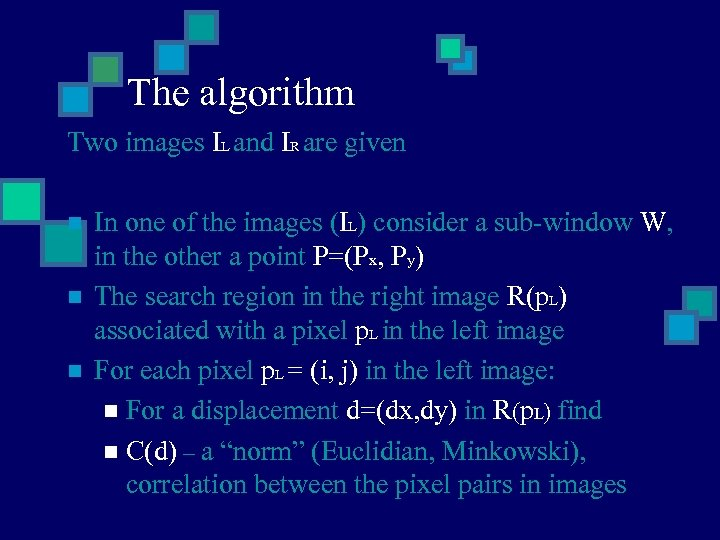The algorithm Two images IL and IR are given n In one of the images (IL) consider a sub-window W, in the other a point P=(Px, Py) The search region in the right image R(p. L) associated with a pixel p. L in the left image For each pixel p. L = (i, j) in the left image: n For a displacement d=(dx, dy) in R(p. L) find n C(d) – a “norm” (Euclidian, Minkowski), correlation between the pixel pairs in imagesn Example: I choose the absolute difference between RGB pixel values: C(d) = Sum. Abs(PV (Wij)-PV (IR(Px+i+dx), IR(Py+j+dy))) This expresses that we count the difference The disparity of p. L is the vector d’=(dx’, dy’) that minimizes C(d) over R(pr) d’ = arg min[C(d)] Improvements: I used edge-define in each picture, to produce more accurate results, given that I work with colored pictures in RGB space, so the algorithm is more like a feature-based algorithm without rotation and stretching (R, G, B) n n (R, G, B)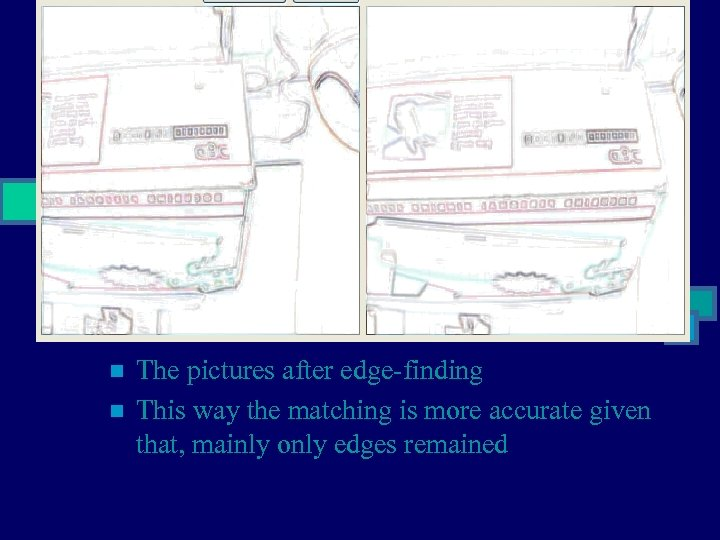n n The pictures after edge-finding This way the matching is more accurate given that, mainly only edges remained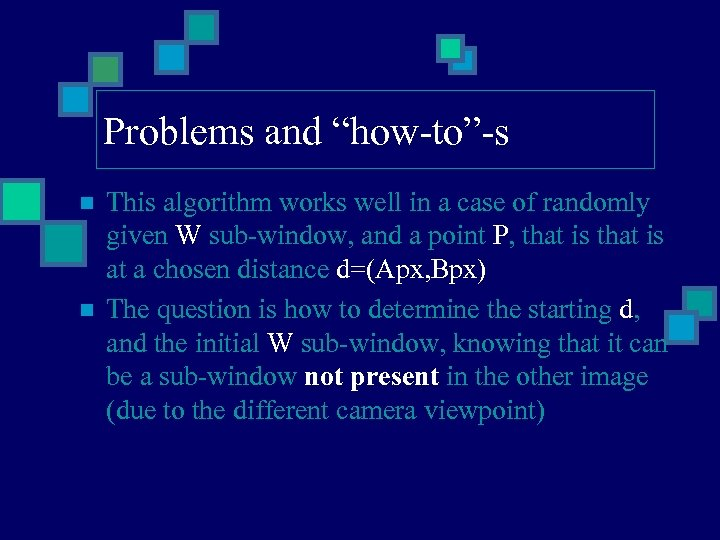Problems and “how-to”-s n n This algorithm works well in a case of randomly given W sub-window, and a point P, that is at a chosen distance d=(Apx, Bpx) The question is how to determine the starting d, and the initial W sub-window, knowing that it can be a sub-window not present in the other image (due to the different camera viewpoint)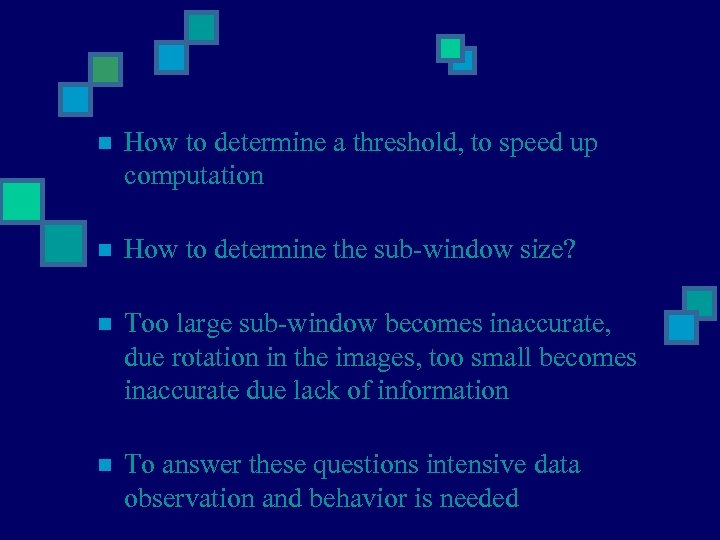n How to determine a threshold, to speed up computation n How to determine the sub-window size? n Too large sub-window becomes inaccurate, due rotation in the images, too small becomes inaccurate due lack of information n To answer these questions intensive data observation and behavior is needed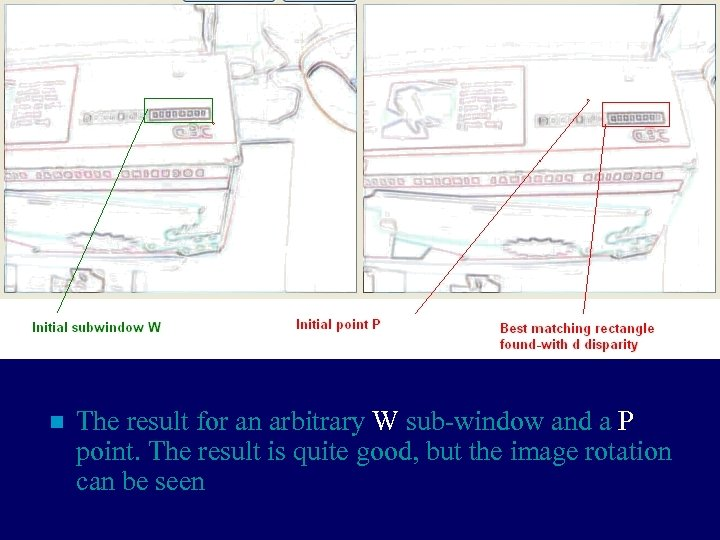n The result for an arbitrary W sub-window and a P point. The result is quite good, but the image rotation can be seen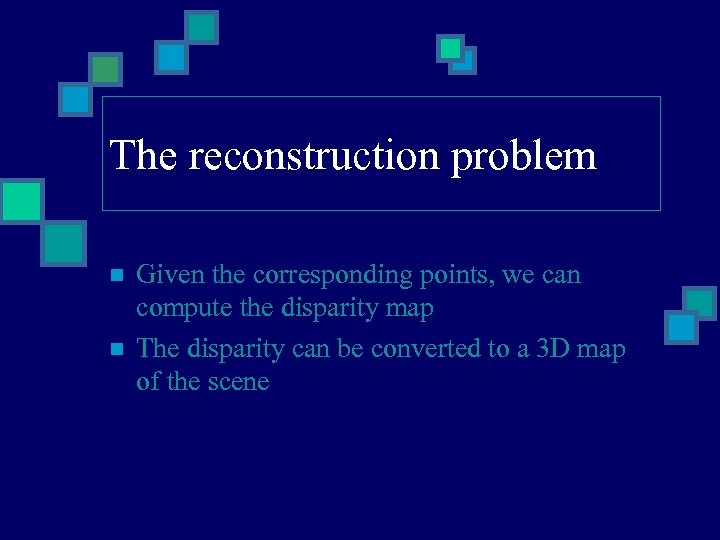The reconstruction problem n n Given the corresponding points, we can compute the disparity map The disparity can be converted to a 3 D map of the scene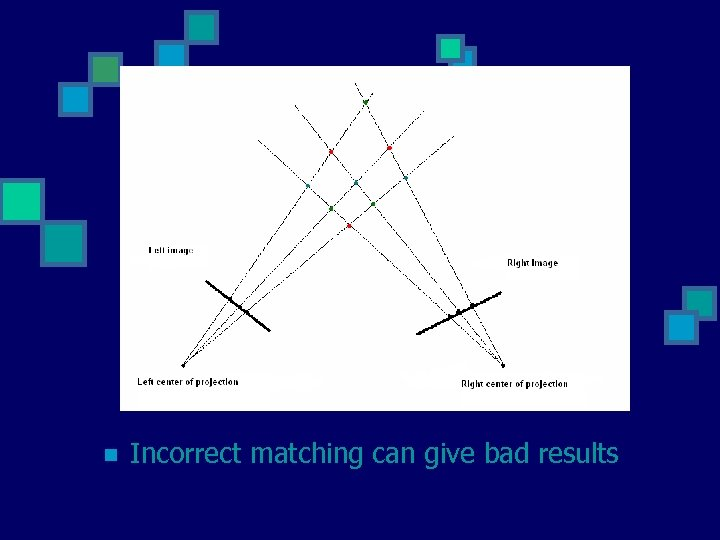n Incorrect matching can give bad results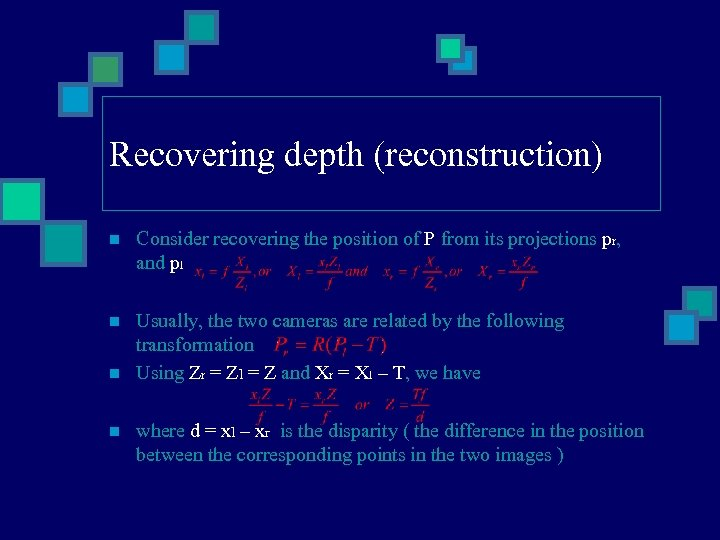Recovering depth (reconstruction) n Consider recovering the position of P from its projections pr, and pl n Usually, the two cameras are related by the following transformation Using Zr = Zl = Z and Xr = Xl – T, we have n n where d = xl – xr is the disparity ( the difference in the position between the corresponding points in the two images )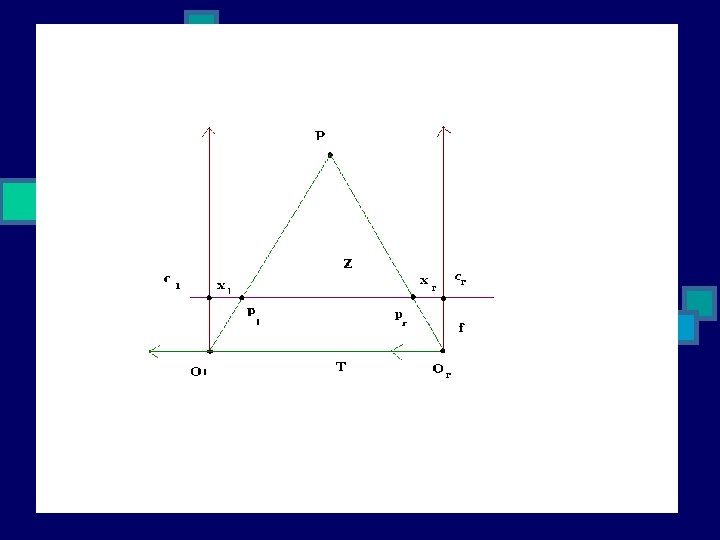The camera is set on an old printer, that helps it moving in the same plane. This improves the stereo vision with single camera moving in a plane.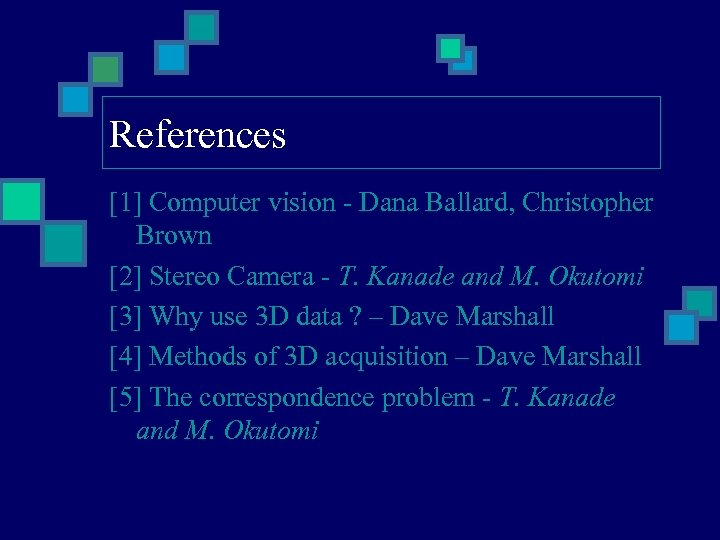References  Computer vision - Dana Ballard, Christopher Brown  Stereo Camera - T. Kanade and M. Okutomi  Why use 3 D data ? – Dave Marshall  Methods of 3 D acquisition – Dave Marshall  The correspondence problem - T. Kanade and M. Okutomi